# Introduction

In social science (and educational) research, we often wish to understand how robust inferences about effects are to unobserved (or controlled for) covariates, possible problems with measurement, and other sources of bias. The goal of konfound is to carry out sensitivity analysis to help analysts to quantify how robust inferences are to potential sources of bias. This package provides functions based on developments in sensitivity analysis by Frank and colleagues, which previously have been implemented in Stata and through an Excel spreadsheet, in R through the konfound package. In particular, we provide functions for both values from analyses carried out outside of R as well as from models (lm(), glm(), and lme4::lmer() fit in R) for:

• Quantifying the bias necessary to alter an inference from the framework of Rubin’s (1974) causal model
• The robustness of causal inference in terms of the impact threshold of a confounding variable

You can install konfound with the following:

install.packages("konfound")

You can then load konfound with the library() function:

library(konfound)
#> Sensitivity analysis as described in Frank, Maroulis, Duong, and Kelcey (2013) and in Frank (2000).
#> For more information visit http://konfound-it.com.

## Use of pkonfound() for values from an already-conducted analysis

pkonfound() is used when we have values from an already-conducted analysis (like a regression analysis), such as one in an already-published study or from an analysis carried out using other software.

In the case of a regression analysis, values from the analysis would simply be used as the inputs to the pkonfound() function. For example, in the use below, we simply enter the values for the estimated effect (an unstandardardized beta coefficient) (2), its standard error (.4), the sample size (100), and the number of covariates (3):

pkonfound(2, .4, 100, 3)
#> Robustness of Inference to Replacement (RIR):
#> To invalidate an inference,  60.3 % of the estimate would have to be due to bias.
#> This is based on a threshold of 0.794 for statistical significance (alpha = 0.05).
#>
#> To invalidate an inference,  60  observations would have to be replaced with cases
#> for which the effect is 0 (RIR = 60).
#>
#> See Frank et al. (2013) for a description of the method.
#>
#> Citation: Frank, K.A., Maroulis, S., Duong, M., and Kelcey, B. (2013).
#> What would it take to change an inference?
#> Using Rubin's causal model to interpret the robustness of causal inferences.
#> Education, Evaluation and Policy Analysis, 35 437-460.
#> For other forms of output, run ?pkonfound and inspect the to_return argument
#> For models fit in R, consider use of konfound().

For this set of values, around 60% would need to be false due to a source of bias for the inference to be invalidated (based on statistical significance and a p-value (or alpha) of .05), possible a very robust effect. An omitted, confounding variable (sometimes referred to as a covariate) would need to have an impact (defined as the product of the confounding variable’s correlation with both the predictor of interest and the outcome) of 0.323, presenting a different interpretation of how robust this (hypothetical) effect is to a variable which is important but not included in the analysis.

Here is another example, but one in which the unstandardized beta coefficient is smaller than its standard error:

pkonfound(.4, 2, 100, 3)
#> Robustness of Inference to Replacement (RIR):
#> To sustain an inference,  89.924 % of the estimate would have to be due to bias.
#> This is based on a threshold of 3.97 for statistical significance (alpha = 0.05).
#>
#> To sustain an inference, 90 of the cases with 0 effect would have to be replaced with cases at the threshold of inference (RIR = 90).
#> See Frank et al. (2013) for a description of the method.
#>
#> Citation: Frank, K.A., Maroulis, S., Duong, M., and Kelcey, B. (2013).
#> What would it take to change an inference?
#> Using Rubin's causal model to interpret the robustness of causal inferences.
#> Education, Evaluation and Policy Analysis, 35 437-460.
#> For other forms of output, run ?pkonfound and inspect the to_return argument
#> For models fit in R, consider use of konfound().

Note that this use of pkonfound() is equivalent to naming the arguments, i.e. for a different set of values:

pkonfound(est_eff = -2.2,
std_err = .65,
n_obs = 200,
n_covariates = 3)
#> Robustness of Inference to Replacement (RIR):
#> To invalidate an inference,  41.732 % of the estimate would have to be due to bias.
#> This is based on a threshold of -1.282 for statistical significance (alpha = 0.05).
#>
#> To invalidate an inference,  83  observations would have to be replaced with cases
#> for which the effect is 0 (RIR = 83).
#>
#> See Frank et al. (2013) for a description of the method.
#>
#> Citation: Frank, K.A., Maroulis, S., Duong, M., and Kelcey, B. (2013).
#> What would it take to change an inference?
#> Using Rubin's causal model to interpret the robustness of causal inferences.
#> Education, Evaluation and Policy Analysis, 35 437-460.
#> For other forms of output, run ?pkonfound and inspect the to_return argument
#> For models fit in R, consider use of konfound().

We notice that the output includes a message that says we can view other forms of output by changing the to_return argument. Here are the two plots - for the bias necessary to alter an inference (thresh_plot) and for the robustness of an inference in terms of the impact of a confounding variable (corr_plot) that can be returned:

pkonfound(.4, 2, 100, 3, to_return = "thresh_plot")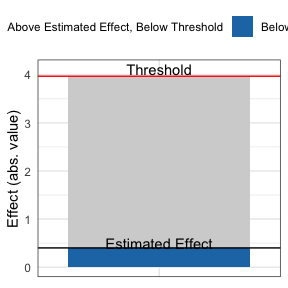pkonfound(.4, 2, 100, 3, to_return = "corr_plot")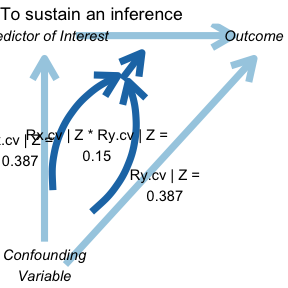You can also specify multiple forms of output at once.

model_output <- pkonfound(2, .4, 200, 3, to_return = c("raw_output", "thresh_plot", "corr_plot"))
#> Robustness of Inference to Replacement (RIR):
#> To invalidate an inference,  60.557 % of the estimate would have to be due to bias.
#> This is based on a threshold of 0.789 for statistical significance (alpha = 0.05).
#>
#> To invalidate an inference,  121  observations would have to be replaced with cases
#> for which the effect is 0 (RIR = 121).
#>
#> See Frank et al. (2013) for a description of the method.
#>
#> Citation: Frank, K.A., Maroulis, S., Duong, M., and Kelcey, B. (2013).
#> What would it take to change an inference?
#> Using Rubin's causal model to interpret the robustness of causal inferences.
#> Education, Evaluation and Policy Analysis, 35 437-460.
#> Print output created by default. Created 3 other forms of output. Use list indexing or run summary() on the output to see how to access.
summary(model_output)
#> Created 3 forms of output. To access type:
#>
#> model_output$raw_output #> model_output$thresh_plot
#> model_output$corr_plot When we type the name of the object, we see that we created three types of output that we can access as follows: model_output$raw_output
#> # A tibble: 1 x 8
#>   action  inference percent_bias_to… replace_null_ca… unstd_beta beta_threshhold
#>   <chr>   <chr>                <dbl>            <dbl>      <dbl>           <dbl>
#> 1 to_inv… reject_n…             60.6              121          2           0.789
#> # … with 2 more variables: omitted_variable_corr <dbl>, itcv <dbl>
model_output$thresh_plot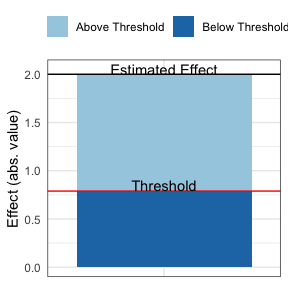model_output$corr_plot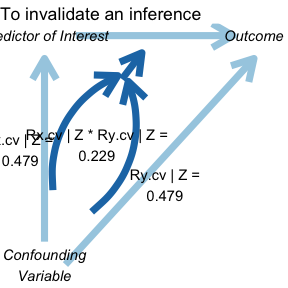Finally, you can return the raw output, for use in other analyses.

pkonfound(.4, 2, 100, 3, to_return = "raw_output")
#> # A tibble: 1 x 8
#>   action  inference percent_bias_to… replace_null_ca… unstd_beta beta_threshhold
#>   <chr>   <chr>                <dbl>            <dbl>      <dbl>           <dbl>
#> 1 to_sus… fail_to_…             89.9               90        0.4            3.97
#> # … with 2 more variables: omitted_variable_corr <dbl>, itcv <dbl>

This function can be used with the values from a two-by-two table associated with an intervention (represented as a dichotomous predictor variable) that is related with a binary outcome, such as one that could be modeled using a logistic regression. Below:

• a represents an unsuccessful control group outcome
• b represents a successful control group outcome
• c represents an unsuccessful treatment group outcome
• d represents a successful treatment group outcome
pkonfound(a = 35, b = 17, c = 17, d = 38)
#> Background Information:
#> This function calculates the number of cases that would have to be replaced
#> with no effect cases (RIR)to invalidate an inference made about the association
#> between the rows and columns in a 2x2 table.
#> One can also interpret this as switches from one cell to another, such as from
#> the treatment success cell to the treatment failure cell.
#>
#> Conclusion:
#> To invalidate the inference, you would need to replace 13 treatment success
#> cases with null hypothesis cases (RIR = 13).
#> This is equivalent to transferring 9 cases from treatment success to treatment failure.
#> For the User-entered Table, we have an estimated odds ratio of 4.530, with p-value of 0.000:
#>
#> User-entered Table:
#>           Fail Success
#> Control     35      17
#> Treatment   17      38
#>
#>
#> For the Transfer Table, we have an estimated odds ratio of 2.278, with p-value of 0.051:
#> Transfer Table:
#>           Fail Success
#> Control     35      17
#> Treatment   26      29
#>
#> RIR:
#> RIR = 13
#> For other forms of output, run ?pkonfound and inspect the to_return argument
#> For models fit in R, consider use of konfound().

A table can also be passed to this function:

my_table <- tibble::tribble(
~unsuccess, ~success,
35,         17,
17,         38,
)

pkonfound(two_by_two_table = my_table)
#> Background Information:
#> This function calculates the number of cases that would have to be replaced
#> with no effect cases (RIR)to invalidate an inference made about the association
#> between the rows and columns in a 2x2 table.
#> One can also interpret this as switches from one cell to another, such as from
#> the treatment success cell to the treatment failure cell.
#>
#> Conclusion:
#> To invalidate the inference, you would need to replace 13 treatment success
#> cases with null hypothesis cases (RIR = 13).
#> This is equivalent to transferring 9 cases from treatment success to treatment failure.
#> For the User-entered Table, we have an estimated odds ratio of 4.530, with p-value of 0.000:
#>
#> User-entered Table:
#>           Fail Success
#> Control     35      17
#> Treatment   17      38
#>
#>
#> For the Transfer Table, we have an estimated odds ratio of 2.278, with p-value of 0.051:
#> Transfer Table:
#>           Fail Success
#> Control     35      17
#> Treatment   26      29
#>
#> RIR:
#> RIR = 13
#> For other forms of output, run ?pkonfound and inspect the to_return argument
#> For models fit in R, consider use of konfound().

## Use of konfound() for models fit in R

Where pkonfound() can be used with values from already-conducted analyses, konfound() can be used with models (lm(), glm(), and lme4::lmer()) fit in R.

For linear models fit with lm()

m1 <- lm(mpg ~ wt + hp + qsec, data = mtcars)
m1
#>
#> Call:
#> lm(formula = mpg ~ wt + hp + qsec, data = mtcars)
#>
#> Coefficients:
#> (Intercept)           wt           hp         qsec
#>    27.61053     -4.35880     -0.01782      0.51083

konfound(m1, hp)
#> Robustness of Inference to Replacement (RIR):
#> To sustain an inference,  41.923 % of the estimate would have to be due to bias.
#> This is based on a threshold of -0.031 for statistical significance (alpha = 0.05).
#>
#> To sustain an inference, 13 of the cases with 0 effect would have to be replaced with cases at the threshold of inference (RIR = 13).
#> See Frank et al. (2013) for a description of the method.
#>
#> Citation: Frank, K.A., Maroulis, S., Duong, M., and Kelcey, B. (2013).
#> What would it take to change an inference?
#> Using Rubin's causal model to interpret the robustness of causal inferences.
#> Education, Evaluation and Policy Analysis, 35 437-460.
#> For more detailed output, consider setting to_return to table
#> To consider other predictors of interest, consider setting test_all to TRUE.

Like with pkonfound(), we can also output multiple forms of output at once with konfound():

konfound_output <- konfound(m1, hp, to_return = c("raw_output", "thresh_plot", "corr_plot"))
#> Robustness of Inference to Replacement (RIR):
#> To sustain an inference,  41.923 % of the estimate would have to be due to bias.
#> This is based on a threshold of -0.031 for statistical significance (alpha = 0.05).
#>
#> To sustain an inference, 13 of the cases with 0 effect would have to be replaced with cases at the threshold of inference (RIR = 13).
#> See Frank et al. (2013) for a description of the method.
#>
#> Citation: Frank, K.A., Maroulis, S., Duong, M., and Kelcey, B. (2013).
#> What would it take to change an inference?
#> Using Rubin's causal model to interpret the robustness of causal inferences.
#> Education, Evaluation and Policy Analysis, 35 437-460.
#> Print output created by default. Created 3 other forms of output. Use list indexing or run summary() on the output to see how to access.
summary(konfound_output)
#> Created 3 forms of output. To access type:
#>
#> konfound_output$raw_output #> konfound_output$thresh_plot
#> konfound_output$corr_plot Again, we can type each of those, i.e.: konfound_output$raw_output
#> # A tibble: 1 x 8
#>   action  inference percent_bias_to… replace_null_ca… unstd_beta beta_threshhold
#>   <chr>   <chr>                <dbl>            <dbl>      <dbl>           <dbl>
#> 1 to_sus… fail_to_…             41.9               13    -0.0178         -0.0307
#> # … with 2 more variables: omitted_variable_corr <dbl>, itcv <dbl>
konfound_output$thresh_plot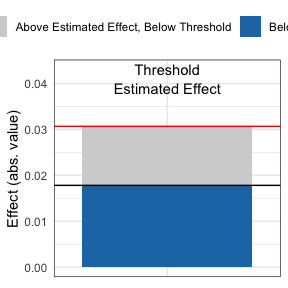We can also test all of the variables as predictors of interest: konfound(m1, wt, test_all = TRUE) #> Note that this output is calculated based on the correlation-based approach used in mkonfound() #> Warning in data.frame(t = est_eff/std_err, df = (n_obs - n_covariates - : row #> names were found from a short variable and have been discarded #> # A tibble: 3 x 8 #> var_name t df action inference pct_bias_to_change_… itcv r_con #> <chr> <dbl> <dbl> <chr> <chr> <dbl> <dbl> <dbl> #> 1 wt -5.79 28 to_inval… reject_null 51.1 0.59 0.768 #> 2 hp -1.19 28 to_susta… fail_to_reje… 39.2 -0.104 0.323 #> 3 qsec 1.16 28 to_susta… fail_to_reje… 40.5 -0.108 0.328 Whereas this cannot be carried out with pkonfound(), with konfound() you can also return a table with some key output from the correlation-based approach. konfound(m1, wt, to_return = "table") #> Dependent variable is mpg #> Warning: Unknown or uninitialised column: itcv. #> Warning: Unknown or uninitialised column: impact. #> # A tibble: 4 x 7 #> term estimate std.error statistic p.value itcv impact #> <chr> <dbl> <dbl> <dbl> <dbl> <dbl> <dbl> #> 1 (Intercept) 27.6 8.42 3.28 0.003 NA NA #> 2 wt -4.36 0.753 -5.79 0 0.602 NA #> 3 hp -0.018 0.015 -1.19 0.244 NA 0.511 #> 4 qsec 0.511 0.439 1.16 0.255 NA 0.073 If the impact threshhold is greater than the impacts of the Zs (the other covariates) then an omitted variable would have to have a greater impact than any of the observed covariates to change the inference. Note that in fields in which there is a lot known about covariates given the outcome of interest, then the omitted ones are likely less important than those that are known an included (i.e., we have a good sense of the factors that matter in terms of educational achievement). For logistic regression models fit with glm() Effects for these models are interpreted on the basis of average partial (or marginal) effects (calculated using the margins package). # if forcats is not installed, this install it first using install.packages("forcats") for this to run if (requireNamespace("forcats")) { d <- forcats::gss_cat d$married <- ifelse(d\$marital == "Married", 1, 0)

m2 <- glm(married ~ age, data = d, family = binomial(link = "logit"))
konfound(m2, age)
}
#> Robustness of Inference to Replacement (RIR):
#> To invalidate an inference,  35.357 % of the estimate would have to be due to bias.
#> This is based on a threshold of 0.002 for statistical significance (alpha = 0.05).
#>
#> To invalidate an inference,  7569  observations would have to be replaced with cases
#> for which the effect is 0 (RIR = 7569).
#>
#> See Frank et al. (2013) for a description of the method.
#>
#> Citation: Frank, K.A., Maroulis, S., Duong, M., and Kelcey, B. (2013).
#> What would it take to change an inference?
#> Using Rubin's causal model to interpret the robustness of causal inferences.
#> Education, Evaluation and Policy Analysis, 35 437-460.
#> NULL

For logistic regression models fit with glm() with a dichotomous predictor of interest

m4 <- glm(outcome ~ condition, data = binary_dummy_data)
konfound(m4, condition, two_by_two = TRUE)

As with models fit with lm() (and use of pkonfound()), multiple forms of output can be specified with the to_return argument to konfound(), i.e. konfound(m2, age, to_return = c("raw_output", "corr_plot", "thresh_plot")).

For mixed effects (or multi-level) models fit with the lmer() function from the lme4 package

konfound also works with models fit with the lmer() function from the package lme4, for mixed-effects or multi-level models. One challenge with carrying out sensitivity analysis for fixed effects in mixed effects models is calculating the correct denominator degrees of freedom for the t-test associated with the coefficients. This is not unique to sensitivity analysis, as, for example, lmer() does not report degrees of freedom (or p-values) for fixed effects predictors (see this information in the lme4 FAQ here). While it may be possible to determine the correct degrees of freedom for some models (i.e., models with relatively simple random effects structures), it is difficult to generalize this approach, and so in this package the Kenward-Roger approximation for the denominator degrees of freedom as implemented in the pbkrtest package (described in Halekoh and Højsgaard, 2014).

Here is an example of the use of konfound() with a model fit with lmer():

if (requireNamespace("lme4")) {
library(lme4)
m3 <- fm1 <- lmer(Reaction ~ Days + (1 | Subject), sleepstudy)
konfound(m3, Days)
}
#> Warning in checkMatrixPackageVersion(): Package version inconsistency detected.
#> TMB was built with Matrix version 1.3.2
#> Current Matrix version is 1.2.18
#> Please re-install 'TMB' from source using install.packages('TMB', type = 'source') or ask CRAN for a binary version of 'TMB' matching CRAN's 'Matrix' package
#> Robustness of Inference to Replacement (RIR):
#> To invalidate an inference,  84.826 % of the estimate would have to be due to bias.
#> This is based on a threshold of 1.588 for statistical significance (alpha = 0.05).
#>
#> To invalidate an inference,  137  observations would have to be replaced with cases
#> for which the effect is 0 (RIR = 137).
#>
#> See Frank et al. (2013) for a description of the method.
#>
#> Citation: Frank, K.A., Maroulis, S., Duong, M., and Kelcey, B. (2013).
#> What would it take to change an inference?
#> Using Rubin's causal model to interpret the robustness of causal inferences.
#> Education, Evaluation and Policy Analysis, 35 437-460.
#> Note that the Kenward-Roger approximation is used to estimate degrees of freedom for the predictor(s) of interest. We are presently working to add other methods for calculating the degrees of freedom for the predictor(s) of interest. If you wish to use other methods now, consider those detailed here: https://bbolker.github.io/mixedmodels-misc/glmmFAQ.html#why-doesnt-lme4-display-denominator-degrees-of-freedomp-values-what-other-options-do-i-have. You can then enter degrees of freedom obtained from another method along with the coefficient, number of observations, and number of covariates to the pkonfound() function to quantify the robustness of the inference.
#> NULL

## Use of mkonfound() for meta-analyses that include sensitivity analysis

We can also use konfound to carry out sensitivity analysis as part of meta-analyses. For example, here, d represents output from a number (30 in this case) of past studies, read in a CSV file from a website:

d <- read.csv("https://msu.edu/~kenfrank/example%20dataset%20for%20mkonfound.csv")
mkonfound(d, t, df)

We can also return a plot summarizing the percent bias needed to sustan or invalidate an inference across all of the past studies:

mkonfound(d, t, df, return_plot = T)

# Other information

To learn more about sensitivity analysis, please visit: ’/ ?˘* The Introduction to konfound vignette, with detailed information about each of the functions (pkonfound(), konfound(), and mkounfound()) * The causal inference section of Ken Frank’s website here * The konfound interactive web application, with links to PowerPoints and key publications
konfound is actively under development as of January, 2018. We welcome feedback and requests for improvement. We prefer for issues to be filed via GitHub (link to the issues page for konfound here) though we also welcome questions or feedback via email (see the DESCRIPTION file).